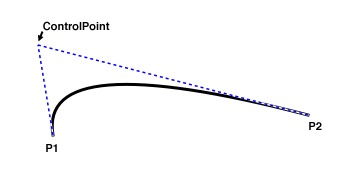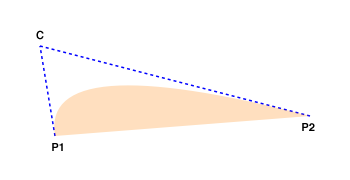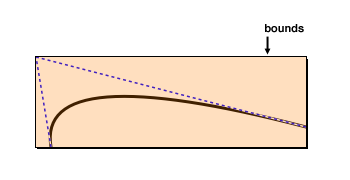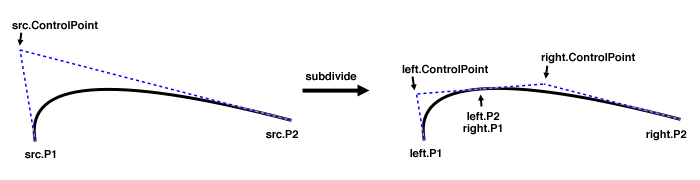# Class QuadCurve2D

• `java.lang.Object`
• `java.awt.geom.QuadCurve2D`
Implemented Interfaces:
Cloneable, Shape
Known Direct Subclasses:
QuadCurve2D.Double, QuadCurve2D.Float

`public abstract class QuadCurve2D`
`extends Object`
`implements Shape, Cloneable`

A two-dimensional curve that is parameterized with a quadratic function.Since:
1.2

## Nested Class Summary

`static class`
`QuadCurve2D.Double`
A two-dimensional curve that is parameterized with a quadratic function and stores coordinate values in double-precision floating-point format.
`static class`
`QuadCurve2D.Float`
A two-dimensional curve that is parameterized with a quadratic function and stores coordinate values in single-precision floating-point format.

## Constructor Summary

`QuadCurve2D()`
Constructs a new QuadCurve2D.

## Method Summary

` Object`
`clone()`
Creates a new curve with the same contents as this one.
` boolean`
`contains(double x, double y)`
Determines whether a point is inside the area bounded by the curve and the straight line connecting its end points.
` boolean`
`contains(double x, double y, double w, double h)`
Determines whether a rectangle is entirely inside the area bounded by the curve and the straight line connecting its end points.
` boolean`
`contains(Point2D p)`
Determines whether a point is inside the area bounded by the curve and the straight line connecting its end points.
` boolean`
`contains(Rectangle2D r)`
Determines whether a Rectangle2D is entirely inside the area that is bounded by the curve and the straight line connecting its end points.
` Rectangle`
`getBounds()`
Determines the smallest rectangle that encloses the curve’s start, end and control point.
`abstract Point2D`
`getCtrlPt()`
Returns the curve’s control point.
`abstract double`
`getCtrlX()`
Returns the x coordinate of the curve’s control point.
`abstract double`
`getCtrlY()`
Returns the y coordinate of the curve’s control point.
` double`
`getFlatness()`
Calculates the flatness of this curve.
`static double`
`getFlatness(double x1, double y1, double cx, double cy, double x2, double y2)`
Calculates the flatness of a quadratic curve, directly specifying each coordinate value.
`static double`
`getFlatness(double[] coords, int offset)`
Calculates the flatness of a quadratic curve, specifying the coordinate values in an array.
` double`
`getFlatnessSq()`
Calculates the squared flatness of this curve.
`static double`
`getFlatnessSq(double x1, double y1, double cx, double cy, double x2, double y2)`
Calculates the squared flatness of a quadratic curve, directly specifying each coordinate value.
`static double`
`getFlatnessSq(double[] coords, int offset)`
Calculates the squared flatness of a quadratic curve, specifying the coordinate values in an array.
`abstract Point2D`
`getP1()`
Returns the curve’s start point.
`abstract Point2D`
`getP2()`
Returns the curve’s end point.
` PathIterator`
`getPathIterator(AffineTransform at)`
Return an iterator along the shape boundary.
` PathIterator`
`getPathIterator(AffineTransform at, double flatness)`
Return an iterator along the flattened version of the shape boundary.
`abstract double`
`getX1()`
Returns the x coordinate of the curve’s start point.
`abstract double`
`getX2()`
Returns the x coordinate of the curve’s end point.
`abstract double`
`getY1()`
Returns the y coordinate of the curve’s start point.
`abstract double`
`getY2()`
Returns the y coordinate of the curve’s end point.
` boolean`
`intersects(double x, double y, double w, double h)`
Determines whether any part of a rectangle is inside the area bounded by the curve and the straight line connecting its end points.
` boolean`
`intersects(Rectangle2D r)`
Determines whether any part of a Rectangle2D is inside the area bounded by the curve and the straight line connecting its end points.
`abstract void`
`setCurve(double x1, double y1, double cx, double cy, double x2, double y2)`
Changes the curve geometry, separately specifying each coordinate value.
` void`
`setCurve(double[] coords, int offset)`
Changes the curve geometry, passing coordinate values in an array.
` void`
`setCurve(Point2D p1, Point2D c, Point2D p2)`
Changes the curve geometry, specifying coordinate values in separate Point objects.
` void`
`setCurve(Point2D[] pts, int offset)`
Changes the curve geometry, specifying coordinate values in an array of Point objects.
` void`
`setCurve(QuadCurve2D c)`
Changes the geometry of the curve to that of another curve.
`static int`
`solveQuadratic(double[] eqn)`
Finds the non-complex roots of a quadratic equation, placing the results into the same array as the equation coefficients.
`static int`
`solveQuadratic(double[] eqn, double[] res)`
Finds the non-complex roots of a quadratic equation.
`static void`
`subdivide(double[] src, int srcOff, double[] left, int leftOff, double[] right, int rightOff)`
Subdivides a quadratic curve into two halves, passing all coordinates in an array.
` void`
`subdivide(QuadCurve2D left, QuadCurve2D right)`
Subdivides this curve into two halves.
`static void`
`subdivide(QuadCurve2D src, QuadCurve2D left, QuadCurve2D right)`
Subdivides a quadratic curve into two halves.

### Methods inherited from class java.lang.Object

`clone`, `equals`, `extends Object> getClass`, `finalize`, `hashCode`, `notify`, `notifyAll`, `toString`, `wait`, `wait`, `wait`

## Constructor Details

### QuadCurve2D

`protected QuadCurve2D()`
Constructs a new QuadCurve2D. Typical users will want to construct instances of a subclass, such as `QuadCurve2D.Float` or `QuadCurve2D.Double`.

## Method Details

### clone

`public Object clone()`
Creates a new curve with the same contents as this one.
Overrides:
clone in interface Object
Returns:
the clone.

### contains

```public boolean contains(double x,
double y)```
Determines whether a point is inside the area bounded by the curve and the straight line connecting its end points.The above drawing illustrates in which area points are considered “inside” a QuadCurve2D.

Specified by:
contains in interface Shape

### contains

```public boolean contains(double x,
double y,
double w,
double h)```
Determines whether a rectangle is entirely inside the area bounded by the curve and the straight line connecting its end points.The above drawing illustrates in which area points are considered “inside” a QuadCurve2D.

Specified by:
contains in interface Shape

### contains

`public boolean contains(Point2D p)`
Determines whether a point is inside the area bounded by the curve and the straight line connecting its end points.The above drawing illustrates in which area points are considered “inside” a QuadCurve2D.

Specified by:
contains in interface Shape

### contains

`public boolean contains(Rectangle2D r)`
Determines whether a Rectangle2D is entirely inside the area that is bounded by the curve and the straight line connecting its end points.
Specified by:
contains in interface Shape

### getBounds

`public Rectangle getBounds()`
Determines the smallest rectangle that encloses the curve’s start, end and control point. As the illustration below shows, the invisible control point may cause the bounds to be much larger than the area that is actually covered by the curve.Specified by:
getBounds in interface Shape

### getCtrlPt

`public abstract Point2D getCtrlPt()`
Returns the curve’s control point.

### getCtrlX

`public abstract double getCtrlX()`
Returns the x coordinate of the curve’s control point.

### getCtrlY

`public abstract double getCtrlY()`
Returns the y coordinate of the curve’s control point.

### getFlatness

`public double getFlatness()`
Calculates the flatness of this curve. The flatness is the distance of the control point to the line between start and end point.In the above drawing, the straight line connecting start point P1 and end point P2 is depicted in gray. The result will be the the distance between C and the gray line, i.e. the length of the red line.

### getFlatness

```public static double getFlatness(double x1,
double y1,
double cx,
double cy,
double x2,
double y2)```
Calculates the flatness of a quadratic curve, directly specifying each coordinate value. The flatness is the distance of the control point to the line between start and end point.In the above drawing, the straight line connecting start point P1 and end point P2 is depicted in gray. The result will be the the distance between C and the gray line, i.e. the length of the red line.

Parameters:
`x1` - the x coordinate of the start point P1.
`y1` - the y coordinate of the start point P1.
`cx` - the x coordinate of the control point C.
`cy` - the y coordinate of the control point C.
`x2` - the x coordinate of the end point P2.
`y2` - the y coordinate of the end point P2.

### getFlatness

```public static double getFlatness(double[] coords,
int offset)```
Calculates the flatness of a quadratic curve, specifying the coordinate values in an array. The flatness is the distance of the control point to the line between start and end point.In the above drawing, the straight line connecting start point P1 and end point P2 is depicted in gray. The result will be the the the distance between C and the gray line, i.e. the length of the red line.

Parameters:
`coords` - an array containing the coordinate values. The x coordinate of the start point P1 is located at `coords[offset]`, its y coordinate at `coords[offset + 1]`. The x coordinate of the control point C is located at `coords[offset + 2]`, its y coordinate at `coords[offset + 3]`. The x coordinate of the end point P2 is located at `coords[offset + 4]`, its y coordinate at `coords[offset + 5]`.
`offset` - the offset of the first coordinate value in `coords`.

### getFlatnessSq

`public double getFlatnessSq()`
Calculates the squared flatness of this curve. The flatness is the distance of the control point to the line between start and end point.In the above drawing, the straight line connecting start point P1 and end point P2 is depicted in gray. The result will be the the square of the distance between C and the gray line, i.e. the squared length of the red line.

### getFlatnessSq

```public static double getFlatnessSq(double x1,
double y1,
double cx,
double cy,
double x2,
double y2)```
Calculates the squared flatness of a quadratic curve, directly specifying each coordinate value. The flatness is the distance of the control point to the line between start and end point.In the above drawing, the straight line connecting start point P1 and end point P2 is depicted in gray. The result will be the the square of the distance between C and the gray line, i.e. the squared length of the red line.

Parameters:
`x1` - the x coordinate of the start point P1.
`y1` - the y coordinate of the start point P1.
`cx` - the x coordinate of the control point C.
`cy` - the y coordinate of the control point C.
`x2` - the x coordinate of the end point P2.
`y2` - the y coordinate of the end point P2.

### getFlatnessSq

```public static double getFlatnessSq(double[] coords,
int offset)```
Calculates the squared flatness of a quadratic curve, specifying the coordinate values in an array. The flatness is the distance of the control point to the line between start and end point.In the above drawing, the straight line connecting start point P1 and end point P2 is depicted in gray. The result will be the the square of the distance between C and the gray line, i.e. the squared length of the red line.

Parameters:
`coords` - an array containing the coordinate values. The x coordinate of the start point P1 is located at `coords[offset]`, its y coordinate at `coords[offset + 1]`. The x coordinate of the control point C is located at `coords[offset + 2]`, its y coordinate at `coords[offset + 3]`. The x coordinate of the end point P2 is located at `coords[offset + 4]`, its y coordinate at `coords[offset + 5]`.
`offset` - the offset of the first coordinate value in `coords`.

### getP1

`public abstract Point2D getP1()`
Returns the curve’s start point.

### getP2

`public abstract Point2D getP2()`
Returns the curve’s end point.

### getPathIterator

`public PathIterator getPathIterator(AffineTransform at)`
Return an iterator along the shape boundary. If the optional transform is provided, the iterator is transformed accordingly. Each call returns a new object, independent from others in use. It is recommended, but not required, that the Shape isolate iterations from future changes to the boundary, and document this fact.
Specified by:
getPathIterator in interface Shape
Parameters:
Returns:
a new iterator over the boundary
Since:
1.2

### getPathIterator

```public PathIterator getPathIterator(AffineTransform at,
double flatness)```
Return an iterator along the flattened version of the shape boundary. Only SEG_MOVETO, SEG_LINETO, and SEG_CLOSE points are returned in the iterator. The flatness parameter controls how far points are allowed to differ from the real curve; although a limit on accuracy may cause this parameter to be enlarged if needed.

If the optional transform is provided, the iterator is transformed accordingly. Each call returns a new object, independent from others in use. It is recommended, but not required, that the Shape isolate iterations from future changes to the boundary, and document this fact.

Specified by:
getPathIterator in interface Shape
Parameters:
`flatness` - the maximum distance for deviation from the real boundary
Returns:
a new iterator over the boundary
Since:
1.2

### getX1

`public abstract double getX1()`
Returns the x coordinate of the curve’s start point.

### getX2

`public abstract double getX2()`
Returns the x coordinate of the curve’s end point.

### getY1

`public abstract double getY1()`
Returns the y coordinate of the curve’s start point.

### getY2

`public abstract double getY2()`
Returns the y coordinate of the curve’s end point.

### intersects

```public boolean intersects(double x,
double y,
double w,
double h)```
Determines whether any part of a rectangle is inside the area bounded by the curve and the straight line connecting its end points.The above drawing illustrates in which area points are considered “inside” in a CubicCurve2D.

Specified by:
intersects in interface Shape

### intersects

`public boolean intersects(Rectangle2D r)`
Determines whether any part of a Rectangle2D is inside the area bounded by the curve and the straight line connecting its end points.
Specified by:
intersects in interface Shape

### setCurve

```public abstract void setCurve(double x1,
double y1,
double cx,
double cy,
double x2,
double y2)```
Changes the curve geometry, separately specifying each coordinate value.
Parameters:
`x1` - the x coordinate of the curve’s new start point.
`y1` - the y coordinate of the curve’s new start point.
`cx` - the x coordinate of the curve’s new control point.
`cy` - the y coordinate of the curve’s new control point.
`x2` - the x coordinate of the curve’s new end point.
`y2` - the y coordinate of the curve’s new end point.

### setCurve

```public void setCurve(double[] coords,
int offset)```
Changes the curve geometry, passing coordinate values in an array.
Parameters:
`coords` - an array containing the new coordinate values. The x coordinate of the new start point is located at `coords[offset]`, its y coordinate at `coords[offset + 1]`. The x coordinate of the new control point is located at `coords[offset + 2]`, its y coordinate at `coords[offset + 3]`. The x coordinate of the new end point is located at `coords[offset + 4]`, its y coordinate at `coords[offset + 5]`.
`offset` - the offset of the first coordinate value in `coords`.

### setCurve

```public void setCurve(Point2D p1,
Point2D c,
Point2D p2)```
Changes the curve geometry, specifying coordinate values in separate Point objects.The curve does not keep any reference to the passed point objects. Therefore, a later change to `p1`, `c` `p2` will not affect the curve geometry.

Parameters:
`p1` - the new start point.
`c` - the new control point.
`p2` - the new end point.

### setCurve

```public void setCurve(Point2D[] pts,
int offset)```
Changes the curve geometry, specifying coordinate values in an array of Point objects.The curve does not keep references to the passed point objects. Therefore, a later change to the `pts` array or any of its elements will not affect the curve geometry.

Parameters:
`pts` - an array containing the points. The new start point is located at `pts[offset]`, the new control point at `pts[offset + 1]`, and the new end point at `pts[offset + 2]`.
`offset` - the offset of the start point in `pts`.

### setCurve

`public void setCurve(QuadCurve2D c)`
Changes the geometry of the curve to that of another curve.
Parameters:
`c` - the curve whose coordinates will be copied.

### solveQuadratic

`public static int solveQuadratic(double[] eqn)`
Finds the non-complex roots of a quadratic equation, placing the results into the same array as the equation coefficients. The following equation is being solved:
`eqn` · x2 + `eqn` · x + `eqn` = 0

For some background about solving quadratic equations, see the article “Quadratic Formula” in PlanetMath. For an extensive library of numerical algorithms written in the C programming language, see the GNU Scientific Library.

Parameters:
`eqn` - an array with the coefficients of the equation. When this procedure has returned, `eqn` will contain the non-complex solutions of the equation, in no particular order.
Returns:
the number of non-complex solutions. A result of 0 indicates that the equation has no non-complex solutions. A result of -1 indicates that the equation is constant (i.e., always or never zero).

### solveQuadratic

```public static int solveQuadratic(double[] eqn,
double[] res)```
Finds the non-complex roots of a quadratic equation. The following equation is being solved:
`eqn` · x2 + `eqn` · x + `eqn` = 0

For some background about solving quadratic equations, see the article “Quadratic Formula” in PlanetMath. For an extensive library of numerical algorithms written in the C programming language, see the GNU Scientific Library.

Parameters:
`eqn` - an array with the coefficients of the equation.
`res` - an array into which the non-complex roots will be stored. The results may be in an arbitrary order. It is safe to pass the same array object reference for both `eqn` and `res`.
Returns:
the number of non-complex solutions. A result of 0 indicates that the equation has no non-complex solutions. A result of -1 indicates that the equation is constant (i.e., always or never zero).

### subdivide

```public static void subdivide(double[] src,
int srcOff,
double[] left,
int leftOff,
double[] right,
int rightOff)```
Subdivides a quadratic curve into two halves, passing all coordinates in an array.The left end point and the right start point will always be identical. Memory-concious programmers thus may want to pass the same array for both `left` and `right`, and set `rightOff` to `leftOff + 4`.

Parameters:
`src` - an array containing the coordinates of the curve to be subdivided. The x coordinate of the start point is located at `src[srcOff]`, its y at `src[srcOff + 1]`. The x coordinate of the control point is located at `src[srcOff + 2]`, its y at `src[srcOff + 3]`. The x coordinate of the end point is located at ```src[srcOff + 4]```, its y at `src[srcOff + 5]`.
`srcOff` - an offset into `src`, specifying the index of the start point’s x coordinate.
`left` - an array that will receive the coordinates of the left half of `src`. It is acceptable to pass `src`. A caller who is not interested in the left half can pass `null`.
`leftOff` - an offset into `left`, specifying the index where the start point’s x coordinate will be stored.
`right` - an array that will receive the coordinates of the right half of `src`. It is acceptable to pass `src` or `left`. A caller who is not interested in the right half can pass `null`.
`rightOff` - an offset into `right`, specifying the index where the start point’s x coordinate will be stored.

### subdivide

```public void subdivide(QuadCurve2D left,
QuadCurve2D right)```
Subdivides this curve into two halves.Parameters:
`left` - a curve whose geometry will be set to the left half of this curve, or `null` if the caller is not interested in the left half.
`right` - a curve whose geometry will be set to the right half of this curve, or `null` if the caller is not interested in the right half.

### subdivide

```public static void subdivide(QuadCurve2D src,
QuadCurve2D left,
QuadCurve2D right)```
Subdivides a quadratic curve into two halves.Parameters:
`src` - the curve to be subdivided.
`left` - a curve whose geometry will be set to the left half of `src`, or `null` if the caller is not interested in the left half.
`right` - a curve whose geometry will be set to the right half of `src`, or `null` if the caller is not interested in the right half.

QuadCurve2D.java -- represents a parameterized quadratic curve in 2-D space Copyright (C) 2002, 2003, 2004 Free Software Foundation This file is part of GNU Classpath. GNU Classpath is free software; you can redistribute it and/or modify it under the terms of the GNU General Public License as published by the Free Software Foundation; either version 2, or (at your option) any later version. GNU Classpath is distributed in the hope that it will be useful, but WITHOUT ANY WARRANTY; without even the implied warranty of MERCHANTABILITY or FITNESS FOR A PARTICULAR PURPOSE. See the GNU General Public License for more details. You should have received a copy of the GNU General Public License along with GNU Classpath; see the file COPYING. If not, write to the Free Software Foundation, Inc., 51 Franklin Street, Fifth Floor, Boston, MA 02110-1301 USA. Linking this library statically or dynamically with other modules is making a combined work based on this library. Thus, the terms and conditions of the GNU General Public License cover the whole combination. As a special exception, the copyright holders of this library give you permission to link this library with independent modules to produce an executable, regardless of the license terms of these independent modules, and to copy and distribute the resulting executable under terms of your choice, provided that you also meet, for each linked independent module, the terms and conditions of the license of that module. An independent module is a module which is not derived from or based on this library. If you modify this library, you may extend this exception to your version of the library, but you are not obligated to do so. If you do not wish to do so, delete this exception statement from your version.